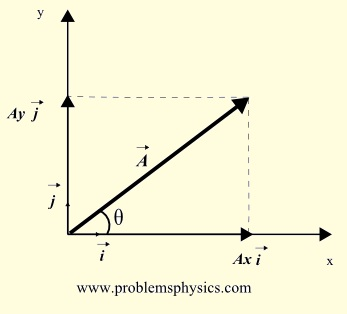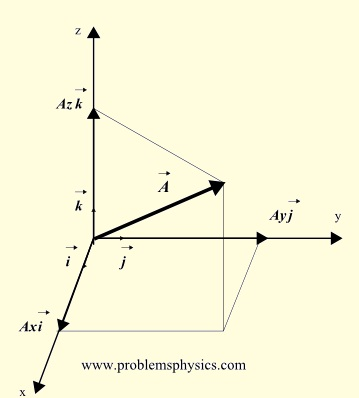# Introduction to Vectors

 IntroductionFig1. - Vector in 2 dimensions. A vector is a mathematical concept to quantify, or describe mathematically, quantities that have a magnitude and a direction. Force, velocity and displacement are examples of quantities that have magnitude and direction and may therefore be described using vectors. Vectors in one dimension describe quantities along one direction and its opposite. Vectors in two dimensions describe quantities in a plane, and vectors in three dimensions describe quantities in 3 dimensional space. 2-dimensional Vectors A two dimensional vector is used to represent a quantity in a plane. On the right, is shown vector A in two dimensions with components Ax and Ay that may written as A→ = Axi + Ayj or A→ = (Ax , Ay) The magnitude of vector A→ is given by | A |→ = √Ax2 + Ay2Fig2. - Vector in 3 dimensions. 3-dimensional Vectors A three dimensional vector is used to represent a quantity in a 3 dimensional space. To the right, is shown vector A in three dimensions with components Ax, Ay and Az that may written as A→ = Axi + Ayj + Azk or A→ = (Ax , Ay , Az) The magnitude of vector A→ is given by | A |→ = √Ax2 + Ay2 + Az2 Magnitude and Direction of 2-dimensional Vector Referring to figure 1 above, θ , the angle between the vector and the positive x-axis direction, in counterclockwise direction, is called the direction of the vector. The relationships between θ, | A |→ and the components Ax and Ay of vector A are: Ax = | A |→ cos (θ) Ay = | A |→ sin (θ) tan(θ)= Ay / Ax Example 1 The magnitude of a 2-dimensional vector is 10 and its direction θ = 135 �. Find its components Ax and Ay. Solution Ax = 10 cos(135�) = 10 (-√2 / 2) = -5 √2 Ay = 10 sin(135�) = 10 √2 / 2 = 5 √2 Example 2 Find the magnitude and direction of the vector B→ = 2i - 2 √3 j Solution |B|→ = √22 + (- 2 √3)2 = 4 tan(θ)= Ay / Ax = - 2 √3 / 2 = - √3 Ignoring the sign, the reference angle to angle θ is equal to arctan(√3) = 60� Since the x-component of the vector is positive and the y-component of the vector is negative, the terminal side of angle θ is in quadrant IV, hence θ = 360� - 60� = 300 � Vector B makes an angle of 300 � with the positive x-axis in counterclockwise direction.